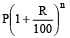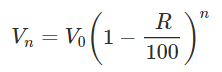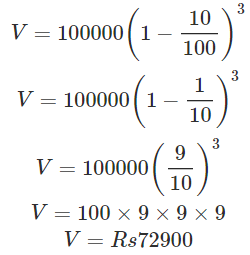Courses

# Points to Remember- Comparing Quantities Class 8 Notes | EduRev

## Mathematics (Maths) Class 8

Created by: Full Circle

## Class 8 : Points to Remember- Comparing Quantities Class 8 Notes | EduRev

The document Points to Remember- Comparing Quantities Class 8 Notes | EduRev is a part of the Class 8 Course Mathematics (Maths) Class 8.
All you need of Class 8 at this link: Class 8

Points to Remember

• For profit, SP > CP and for loss, CP > SP.
• Profit or loss is always calculated on CP.
• The overhead expenses are a part of CP.
• Discount is always calculated on the marked price.
• VAT is not a part of selling price. Of course it is calculated on selling price but it is excluded from the SP while calculating profit or loss.
• Formula for compound interest gives,

Amount =Then CI = A – P.

• If the interest is compounded half yearly the time period becomes twice as much as the number of years.The rate of interest is reduced to half.

We Know That
We compare two quantities by division. Comparing by division is called ratio. Quantities written in ratio have the same units. Ratio has no unit. Two equal ratios are said to form a proportion. In a proportion, product of extremes is equal to the product of the means.

Another way of comparing quantities is to express them as percentages. Percentage means hundredth. It is the numerator of a fraction with denominator 100. To convert ratio into pe cent, we convert it into a fraction with denominator as 100. In unitary method, we find the value for one (i.e. for a unit) and then find the value of many.

Solved Examples:
Question 1: In a survey of 50 students, 80% of students liked Science, and 20% of students liked Arts. How many numbers of students liked Science?
Solution:
We know that 80% of students liked science in the survey of 50 students
∴ Number of students who liked Science = 80/100 × 50 = 40

Question 2: In Yashoda hospital 35 old-aged patients died out of 210 admitted in 2016. In 2017, 10 old-aged patients died out of 150. What’s the increase or decrease of the death rate in the hospital?
Solution:
Here, the base value is different for both the cases. Therefore, converting both the data into a percentage:
Death rate (%) in 2016 = 35/210 × 100 = 16.66%
Death rate (%) in 2017 = 10/150 × 100 = 6.66%
Clearly, there is a decrease in death rate in the hospital in 2017 when compared to that in 2016.
∴ Decrease in death rate (%) = (16.66−6.66)/100 × 100 = 10%

Question 3: The value of a residential flat constructed at a cost of Rs100000 is depreciating at the rate of 10% per annum. What will be its value 3 years after construction?
Solution:
We have: V0= Initial value= Rs 100000, R= Rate of depreciation = 10% per annum, n = 3 years. Therefore: Value after n years,after putting value of n and R, we get:,

,

,

,

,

,

,

,

,

,

,

,

,

,

,

,

,

,

,

,

,

;